Home » FAQ » Can I Run a 60Hz Motor on 50Hz by Using a VFD?

# Can I Run a 60Hz Motor on 50Hz by Using a VFD?

We are often asked “can the motor with a rated frequency of 60Hz operate at 50Hz (vice versa)?” When the motor is operated at a frequency different from the one on the nameplate, the following problems may occur.

Synchronization speed of AC motor depends on two factors: pole number of motor and frequency of motor's input power. For a specific motor, the pole number can’t be changed because it’s determined by the physical structure of motor. The frequency of input power is usually determined by the power distribution system in the country where the motor operates. Commonest frequencies are 50 Hz and 60 Hz.
Reducing the frequency of AC motor will also reduce its speed. On the fan-cooled AC motor, a slower speed may mean that the fan will not turn off the motor. This may mean that the motor’s continuous rated load torque will also be reduced. A slower motor also means that the motor’s rated power would be reduced.

Can the motor with a rated frequency of 60Hz operate at 50Hz? We will study some possible problems that may occur when motor is operated at frequencies different from nameplate frequencies.

Another important parameter that changes with frequency is the ratio of volt to hertz. AC motors are designed to operate at a specific V/Hz ratio, and resistance varies with frequency in AC motors. If the voltage remains constant and the resistance decreases, the current increases. The increased current can provide greater torque, but it can also cause more resistance loss in motors, which may lead to overheat of motor. Similarly, if the voltage remains constant and the resistance increases, the current decreases. Reducing the current means that the motor will have less torque. To avoid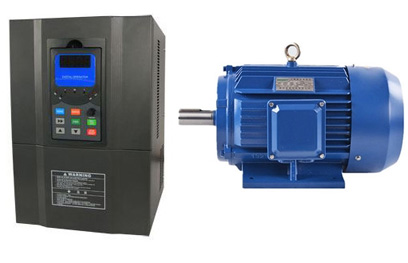above two cases, a constant V / Hz ratio shall be kept.

And this is the working principle of variable frequency drives. Keeping a constant V / Hz ratio through VFD, the current absorbed by the motor remains unchanged and can the motor can maintain torque at different speeds. It also means that the motor with a rated voltage of 240 VAC, 60 Hz (V / Hz = 240/60 = 4) can operate at 200 VAC, 50 Hz (V / Hz = 200/50 = 4).

Whenever possible, the motor with rated operating frequency should be selected. If the motor needs to operate at a higher or lower frequency than the rated frequency, as long as you run the VFD within the maximum allowable frequency range of the motor, there will be no substantial damage, but it may result in losses, depending on the motor parameters.

Plz Calculate (4 - 4) =
(Your comment will show after approved.)

You may also like:Variable Frequency Drives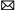inquiry@ato.com.cn

Featured Articles
Variable Frequency Drive for Fans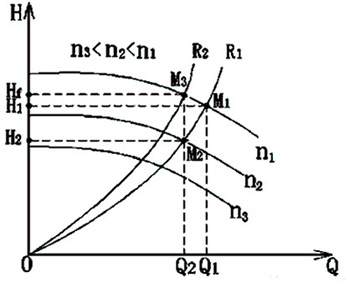Fan is a kind of machine that compresses and conveys gas. If the pressure of exhausted air is quite low, the fan plays a role of ...
Variable Frequency Drive for Food ...Nowadays the food machinery industry has been rapidly developed. As the motor drive, variable frequency drive will change the ...
Can I Run a 60Hz Motor on 50Hz by ...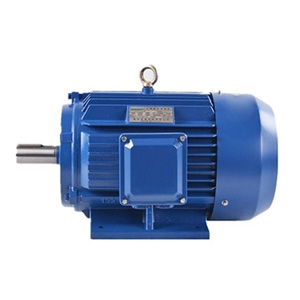We are often asked “can the motor with a rated frequency of 60Hz operate at 50Hz (vice versa)?” When the motor is operated at a ...
How Does Variable Frequency Drive ... The rotating speed of inductor type AC motor (hereinafter referred to as motor) is determined by the number of pole and ...
What is a Variable Frequency Drive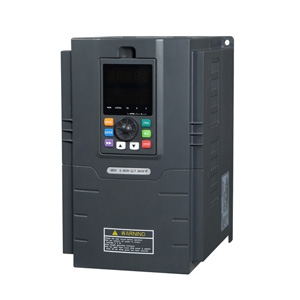Variable frequency drive (VFD) is an adjustable speed drive used for motor drive system, control the speed and torque of the AC ...